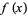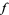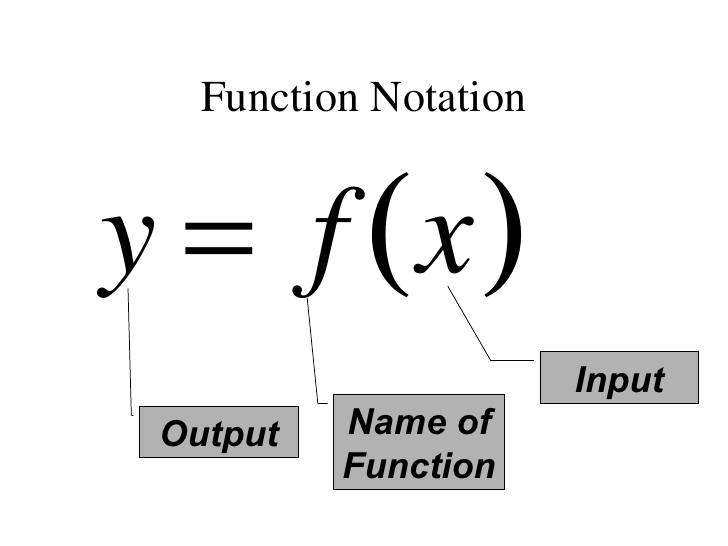# Question about Notation of Function

• B
• SHASHWAT PRATAP SING
In summary, in the notation of Function ---> f(x)=y, f represents the function and x is the variable in the function. We read f(x) as "f of x" or "function of the variable x". The parentheses are used to enclose the variables of a function and signify that the values of the function are dependent upon the values of the variable. The letter y represents the image or output of the function, and is used to abbreviate the notation of the function.

#### SHASHWAT PRATAP SING

In the notation of Function ---> f(x)=y
Here f represents the function and x is the variable in the function.
we read f(x) as "f of x"or "function of the variable x"
what does "function of x " mean that we read ?
in this notation what does round brackets () stands for does that mean "multiply f and x" ?

•Delta2
No it means evaluate ##f## in ##x##.

•S.G. Janssens
Yes, exactly.

Unfortunately, in science texts and older mathematics texts, you will often read things like: "Let ##f(x)## be a function such that..." or the like. The more correct formulation would be: "Let ##f : X \to Y## be a function such that...", where ##X## is the domain and ##Y## is the codomain. In this more correct formulation, you could then write, e.g. "Let ##f : \mathbb{R} \to \mathbb{R}## be defined by ##f(x) := x\sin{x}## for all ##x \in \mathbb{R}##."

While I strongly prefer the more correct formulation, encountering the former is almost unavoidable. So, just read critically and carefully to make sure you understand what is meant.

To the OP, regarding your personal message:
SHASHWAT PRATAP SING said:
I Know f represents our function and x is the variable in the function but then what does the Parentheses () mean.
The parentheses are there just for clarify. Without them, you would write ##fx## instead of ##f(x)##, making it hard to separate the symbol for the function (##f##) from the independent variable (##x##).

(There exist subject areas where the parentheses are actually omitted, but maybe let's not go there now.)

••Delta2 and SHASHWAT PRATAP SING
S.G. Janssens said:
To the OP, regarding your personal message:

The parentheses are there just for clarify. Without them, you would write ##fx## instead of ##f(x)##, making it hard to separate the symbol for the function (##f##) from the independent variable (##x##).

(There exist subject areas where the parentheses are actually omitted, but maybe let's not go there now.)

can we also say that Parentheses are used to enclose the variables of a function in the form, which means that values of the functionare dependent upon the values of.

also sir/maam what does "function of x " mean that we read in the notation---> f(x)=y

S.G. Janssens said:
Unfortunately, in science texts and older mathematics texts, you will often read things like: "Let ##f(x)## be a function such that..." or the like. The more correct formulation would be: "Let ##f : X \to Y## be a function such that...", where ##X## is the domain and ##Y## is the codomain. In this more correct formulation, you could then write, e.g. "Let ##f : \mathbb{R} \to \mathbb{R}## be defined by ##f(x) := x\sin{x}## for all ##x \in \mathbb{R}##."

While I strongly prefer the more correct formulation, encountering the former is almost unavoidable. So, just read critically and carefully to make sure you understand what is meant.
There is another important usage in abstract algebra. ##f(x)\in A[X]## means that ##f(x)## is a polynomial with coefficients in ##A##. It is the abbreviation of the finite sequence ##(f_0,f_1,\ldots,f_n)\in A^n## which represents ##f(x)=f_0+f_1x+\ldots + f_nx^n##. In this case we do not evaluate at ##x## but consider it as single element of ##A[x].## Evaluations such as ##f(a)## with ##a\in A## are distinguished from ##f(x)## in that case.

So notation is always context sensitive, and sometimes even varies from author to author within the same context. It is important to look it up if there are doubts.
SHASHWAT PRATAP SING said:
can we also say that Parentheses are used to enclose the variables of a function in the form View attachment 273300, which means that values of the function View attachment 273301 are dependent upon the values of View attachment 273302.
A function is a special relation, i.e. a subset of the cartesian product ##X\times Y## of domain ##X## and range ##Y##. Instead of ##f\in X\times Y## we write ##f\, : \,X\longrightarrow Y##, and instead of ##(x,y)\in f## we write ##f(x)=y##.
also sir/maam what does "function of x " mean that we read in the notation---> f(x)=y
Not necessarily. If you say "function of x " then you have the first part ##f(x)##. Whether you abbreviate this with an ##y## is another question. You could also abbreviate it with another letter, e.g. ##f(x)=z## or not at all and only operate with ##x## and ##f(x)## as quantities. But if you have ##f(x)=y## then this means: ##f## is a "function of x" whose image we abbreviate with ##y##.

I’m sorry if this is a silly question but I am very confused.

so, I will start from begning-
we represent function by letters f,g,h...etc, and the variable of the function are represented by x,y,...etc.
Thus, the complete representation of function is done by f(x)= RULE . Now The letter y represents the output of the function.
Here, f(x) represents our function, we read f(x) as --> "function of the variable x" meaning we have the Rule as our function and x is the variable in it. thus, this is our complete representation of function with its output.Now, if this is our complete representation of Function along with its output. Then why many people say f(x) and y both represents the output of the function if we represent the [output of the function with y] and [our function with f(x)] then why people mix both the representations ?
if we have seprate representation (y) for the output of the function and and seprate representation f(x) for our function. then why people say f(x) and y both represents the output of the function.

SHASHWAT PRATAP SING said:
I’m sorry if this is a silly question but I am very confused.

so, I will start from begning-
we represent function by letters f,g,h...etc, and the variable of the function are represented by x,y,...etc.
Thus, the complete representation of function is done by f(x)= RULE . Now The letter y represents the output of the function.
Here, f(x) represents our function
, we read f(x) as --> "function of the variable x" meaning we have the Rule as our function and x is the variable in it. thus, this is our complete representation of function with its output.
View attachment 273348
This is not the complete representation, as there is no rule given as to how a y value is produced from a given x value. Other important considerations are the domain -- the set of input values -- and the range -- the set of outputs.
SHASHWAT PRATAP SING said:
Now, if this is our complete representation of Function along with its output. Then why many people say f(x) and y both represents the output of the function if we represent the [output of the function with y] and [our function with f(x)] then why people mix both the representations ?
Because y and f(x) are equal, as is clearly shown in the image above.
SHASHWAT PRATAP SING said:
if we have seprate representation (y) for the output of the function and and seprate representation f(x) for our function. then why people say f(x) and y both represents the output of the function.

•SHASHWAT PRATAP SING
This post went on for way longer than I intended. Possibly some of it will be of use. Very little of what appears below appeared in any textbooks that I can remember or was presented by any teacher. Instead, this sort of stuff is picked up by osmosis having seen proper mathematical usage over the years.

You seem to want to break apart the notation for f(x) = y and attach meaning to each of the pieces individually. As has been pointed out, the meaning can be context sensitive. Sometimes the individual pieces mean something when presented together that cannot be deduced from what they might be taken to mean separately.

That said, I will try to give meaning to the various pieces.

"f" denotes the function itself. Conventionally functions are denoted by the letters f, g or h, but that rule is not sacred. Any letter could be used instead.

Often I would write f() to denote the function. In this case the empty pair of parentheses are there to emphasize that it is a function that is being denoted rather than a number, a set, a vector, a matrix or something else.

"f( something )" denotes the value of the function evaluated at the <something>. So if we have the function f defined so that f(x) = x^2 the f(2) is the number 4.

"x" by itself means nothing. It is simply a convenient variable name. Conventionally the letters x, y, z and w are often used as variable names. Often, x is used as the name of a free variable.

When defining a function, one will often find something like "let f be the function defined by ##f(x) = x^2##". In this context, x acts a placeholder. The right hand side of this equation is expected to be a formula with one free variable. In the case here, that free variable is named "##x##". The ##x## in ##f(x)## tells you which variable in the expression on the right hand side you are choosing.

Note that one will conventionally put the function invocation on the left and the expression used to define it on the right. The left right order is important. It is jarring if written the other way. One wants not to jar the reader.

If, for example, someone said to you, "let f be the function defined by ##f(x) = x + y##" then you would know that you are expected to treat y as a previously defined constant. You could then safely reason that f(4) = f(2) + 2 regardless of what y turns out to be.

On the other hand if someone said "let f be the function defined by ##f(y) = x + y## then you would know that you are expected to treat x as a previously defined constant. You could still safely reason that f(4) = f(2) + 2 regardless of whatever x turns out to be.

If someone (typically a engineer or physicist) says "let ##y = f(x)##", they are probably setting up to make a graph of the function with y on the vertical axis and x on the horizontal. Note that this time we have the function invocation on the right and a variable name on the left. As above, ordering is important. The predefined thing is on the right. The newly defined thing is on the left. There is nothing particularly meaningful about the variable names x and y here except that x is conventionally the independent variable and y conventionally is the dependent variable defined in terms of x. Also, conventionally, the independent variable goes on the horizontal axis and the dependent variable goes on the vertical.

••jim mcnamara, Delta2, SHASHWAT PRATAP SING and 1 other person
jbriggs444 said:
This post went on for way longer than I intended. Possibly some of it will be of use. Very little of what appears below appeared in any textbooks that I can remember or was presented by any teacher. Instead, this sort of stuff is picked up by osmosis having seen proper mathematical usage over the years.

You seem to want to break apart the notation for f(x) = y and attach meaning to each of the pieces individually. As has been pointed out, the meaning can be context sensitive. Sometimes the individual pieces mean something when presented together that cannot be deduced from what they might be taken to mean separately.

That said, I will try to give meaning to the various pieces.

"f" denotes the function itself. Conventionally functions are denoted by the letters f, g or h, but that rule is not sacred. Any letter could be used instead.

Often I would write f() to denote the function. In this case the empty pair of parentheses are there to emphasize that it is a function that is being denoted rather than a number, a set, a vector, a matrix or something else.

"f( something )" denotes the value of the function evaluated at the <something>. So if we have the function f defined so that f(x) = x^2 the f(2) is the number 4.

"x" by itself means nothing. It is simply a convenient variable name. Conventionally the letters x, y, z and w are often used as variable names. Often, x is used as the name of a free variable.

When defining a function, one will often find something like "let f be the function defined by ##f(x) = x^2##". In this context, x acts a placeholder. The right hand side of this equation is expected to be a formula with one free variable. In the case here, that free variable is named "##x##". The ##x## in ##f(x)## tells you which variable in the expression on the right hand side you are choosing.

Note that one will conventionally put the function invocation on the left and the expression used to define it on the right. The left right order is important. It is jarring if written the other way. One wants not to jar the reader.

If, for example, someone said to you, "let f be the function defined by ##f(x) = x + y##" then you would know that you are expected to treat y as a previously defined constant. You could then safely reason that f(4) = f(2) + 2 regardless of what y turns out to be.

On the other hand if someone said "let f be the function defined by ##f(y) = x + y## then you would know that you are expected to treat x as a previously defined constant. You could still safely reason that f(4) = f(2) + 2 regardless of whatever x turns out to be.

If someone (typically a engineer or physicist) says "let ##y = f(x)##", they are probably setting up to make a graph of the function with y on the vertical axis and x on the horizontal. Note that this time we have the function invocation on the right and a variable name on the left. As above, ordering is important. The predefined thing is on the right. The newly defined thing is on the left. There is nothing particularly meaningful about the variable names x and y here except that x is conventionally the independent variable and y conventionally is the dependent variable defined in terms of x. Also, conventionally, the independent variable goes on the horizontal axis and the dependent variable goes on the vertical.

Thanks jbriggs444 for helping me, really it means a lot.SHASHWAT PRATAP SING said:
what does "function of x " mean that we read ?

As an isolated sentence, saying "f is a function of x" has no technical meaning.

For example, the following two sentences define the same function:

Let ##f## be the function ##f(h) = h^2 + 1##
Let ##f## be the function ##f(x) = x^2 + 1##.

It is a cultural tradition to use "x" as the variable when we state the rule that defines a function. It is also a cultural tradition to imagine a function as a graph and use "x" to represent the horizontal coordinate and "y" to represent the vertical coordinate. So, by tradition, we write "y = f(x)" as way of mentioning both coordinates.

In a particular context , the sentence "f is a function of x" may convey technical information. For example if an article has mentioned several different variables x,y,z,..., then the sentence "f is a function of x" informs the reader which of variables is being used to represent the argument (i.e. the input) of the function f.

For example, in a article where "t" represents elapsed time and "x" represents the x-coordinate of an object, the author may write "x is a function of t". In such a situation, there is a reason not to follow the cultural tradition of naming the function "f" and the input "x".

•jbriggs444
SHASHWAT PRATAP SING said:
In the notation of Function ---> f(x)=y
Here f represents the function and x is the variable in the function.
we read f(x) as "f of x"or "function of the variable x"
what does "function of x " mean that we read ?
Not sure but seems you have difficulty understanding the concept of a function. That's not unusual for beginners. I think it may help if you visualize functions as a "transformation" of something. Like if I take 6 apples and make an apple pie. I've transformed them into a pie! I could write that in function notation as:

$$\text{me}(\text{apples})=\text{pie}$$

I've taken the apples (input) and transformed them by the "me" function into a pie. You can write that sort of functional notation with just about everything: 2x4 lumber transformed by me into a shed, numbers 1 and 2 transformed by the "square" function into numbers 1 and 4. And in general, some set called "x" transformed by a function called "f" into a new set called "y" as ##f(x)=y## or say ##\sin(x)=y## with "f" being the sine function and so forth.
•$$\text{me}(\text{apples})=\text{pie}$$